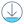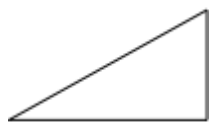# Xamarin.Forms Shapes: PathDownload the sample

The `Path` class derives from the `Shape` class, and can be used to draw curves and complex shapes. These curves and shapes are often described using `Geometry` objects. For information on the properties that the `Path` class inherits from the `Shape` class, see Xamarin.Forms Shapes.

`Path` defines the following properties:

• `Data`, of type `Geometry`, which specifies the shape to be drawn.
• `RenderTransform`, of type `Transform`, which represents the transform that is applied to the geometry of a path prior to it being drawn.

These properties are backed by `BindableProperty` objects, which means that they can be targets of data bindings, and styled.

## Create a Path

To draw a path, create a `Path` object and set its `Data` property. There are two techniques for setting the `Data` property:

• You can set a string value for `Data` in XAML, using path markup syntax. With this approach, the `Path.Data` value is consuming a serialization format for graphics. Typically, you don't edit this string value by hand after it's created. Instead, you use design tools to manipulate the data, and export it as a string fragment that's consumable by the `Data` property.
• You can set the `Data` property to a `Geometry` object. This can be a specific `Geometry` object, or a `GeometryGroup` which acts as a container that can combine multiple geometry objects into a single object.

### Create a Path with path markup syntax

The following XAML example shows how to draw a triangle using path markup syntax:

``````<Path Data="M 10,100 L 100,100 100,50Z"
Stroke="Black"
Aspect="Uniform"
HorizontalOptions="Start" />
``````

The `Data` string begins with the move command, indicated by `M`, which establishes an absolute start point for the path. `L` is the line command, which creates a straight line from the start point to the specified end point. `Z` is the close command, which creates a line that connects the current point to the starting point. The result is a triangle:### Create a Path with Geometry objects

Curves and shapes can be described using `Geometry` objects, which are used to set the `Path` object's `Data` property. There are a variety of `Geometry` objects to choose from. The `EllipseGeometry`, `LineGeometry`, and `RectangleGeometry` classes describe relatively simple shapes. To create more complex shapes or create curves, use a `PathGeometry`.

`PathGeometry` objects are comprised of one or more `PathFigure` objects. Each `PathFigure` object represents a different shape. Each `PathFigure` object is itself comprised of one or more `PathSegment` objects, each representing a connection portion of the shape. Segment types include the following the `LineSegment`, `BezierSegment`, and `ArcSegment` classes.

The following XAML example shows how to draw a triangle using a `PathGeometry` object:

``````<Path Stroke="Black"
Aspect="Uniform"
HorizontalOptions="Start">
<Path.Data>
<PathGeometry>
<PathGeometry.Figures>
<PathFigureCollection>
<PathFigure IsClosed="True"
StartPoint="10,100">
<PathFigure.Segments>
<PathSegmentCollection>
<LineSegment Point="100,100" />
<LineSegment Point="100,50" />
</PathSegmentCollection>
</PathFigure.Segments>
</PathFigure>
</PathFigureCollection>
</PathGeometry.Figures>
</PathGeometry>
</Path.Data>
</Path>
``````

In this example, the start point of the triangle is (10,100). A line segment is drawn from (10,100) to (100,100), and from (100,100) to (100,50). Then the figures first and last segments are connected, because the `PathFigure.IsClosed` property is set to `true`. The result is a triangle: